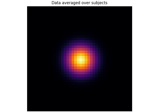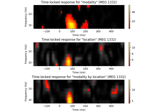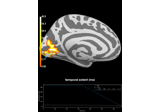# mne.stats.f_mway_rm#

mne.stats.f_mway_rm(data, factor_levels, effects='all', correction=False, return_pvals=True)[source]#

Compute M-way repeated measures ANOVA for fully balanced designs.

Parameters:
data`ndarray`

3D array where the first two dimensions are compliant with a subjects X conditions scheme where the first factor repeats slowest:

```            A1B1 A1B2 A2B1 A2B2
subject 1   1.34 2.53 0.97 1.74
subject ... .... .... .... ....
subject k   2.45 7.90 3.09 4.76
```

The last dimensions is thought to carry the observations for mass univariate analysis.

factor_levelslist-like

The number of levels per factor.

effects

A string denoting the effect to be returned. The following mapping is currently supported (example with 2 factors):

• `'A'`: main effect of A

• `'B'`: main effect of B

• `'A:B'`: interaction effect

• `'A+B'`: both main effects

• `'A*B'`: all three effects

• `'all'`: all effects (equals ‘A*B’ in a 2 way design)

If list, effect names are used: `['A', 'B', 'A:B']`.

correctionbool

The correction method to be employed if one factor has more than two levels. If True, sphericity correction using the Greenhouse-Geisser method will be applied.

return_pvalsbool

If True, return p-values corresponding to F-values.

Returns:
F_vals`ndarray`

An array of F-statistics with length corresponding to the number of effects estimated. The shape depends on the number of effects estimated.

p_vals`ndarray`

If not requested via return_pvals, defaults to an empty array.

Notes

New in version 0.10.

## Examples using `mne.stats.f_mway_rm`#Statistical inference

Statistical inferenceMass-univariate twoway repeated measures ANOVA on single trial power

Mass-univariate twoway repeated measures ANOVA on single trial powerRepeated measures ANOVA on source data with spatio-temporal clustering

Repeated measures ANOVA on source data with spatio-temporal clustering